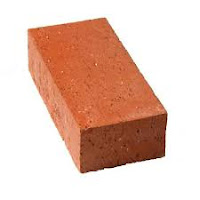# HOW TO CALCULATE CEMENT FOR BRICK WORK ,REQUIRED NO OF BRICKS AND MORTAR (CIVIL ENGINEERING)## SURYA CONSTRUCTION

HOW TO CALCULATE CEMENT FOR BRICK WORK ,REQUIRED NO OF BRICKS  AND MORTAR (CIVIL ENGINEERING TIPS)

In this post, we are going to explain,
• Brickwork Calculation & Formula0’9″ X 0’41/4″ X 0″3″
• How to calculate bricks per square foot
• How to calculate cement quantity & Sand in brickwork

• ## Brickwork BasicsBRICK WALL 4.5″ THICK

Let’s learn the basics,

• Cement mortar ratio should be 1:8 for 9” Brickwork and 1:4 41/2” brickwork
• Mortar thickness should not be more than 10 mm between the courses and sides of the bricks
• The size of the modular brick is 0’9″ X 0’41/4″ X 0″3″ (as per available size)
• Mortar Thickness is 10mm (0.394 Inches)
• Assume required brickwork volume is 100 CFT
Any brick walls consist of bricks and cement mortar.
So, first of all, we are going to find the volume of bricks with mortar thickness and then volume of bricks alone.
Volume of 1 brick with mortar = 0’9″X0’41/4″X0’3″ ( 0.394 inches mortar thickness on all sides)
= 0.0’9.394 X 0’4.64″ X 0’3.394″
Volume of brick with mortar = 0.0864 CFT
Therefore, Number of bricks required for 100 CFT = 100/0.0864 = 1158 No.s

### Volume of bricks without mortar

Volume of 1 brick without mortar = 0’9″ X 0’41/4″ X 0’3″
• Volume of 1 brick without mortar  =  0.0664 CFT
Volume of 500 bricks without mortar = 1157 X 0.0664
Volume of bricks without mortar for 100 CFT = 76.82 CFT (100 CFT)
Therefore,
Required amount of cement mortar = 100 CFT – Volume of bricks without mortar
= 100 – 76.82
Required amount of cement mortar  = 23.18 CFT  (100 CFT) (Wet Condition)
Note – The above volume is in a wet condition that means we need 23.18 cement mortar in mixed condition (after adding water). In order to find the dry volume, we need to multiply 33 % as bulk-age of sand.
Dry volume of a mortar = 23.18 CFT X 1.33 = 30.83 CFT
We know the mortar ratio is 1:8 (1 part Cement & 8 Part Sand = 9 Part)
Required amount Cement quantity in brickwork = 30.83 X 1/7 X 1.25
Volume of cement = 1.25 cft. The reason to multiply this density is, the above multiplication will give us only required amount of cement quantity in brickwork as a cubic feet. But we need cement in bag Therefore we are multiplying the 1.25 cft volume of cement to calculate the cement quantity.
Required amount Cement quantity = 2.74 bgs = 137 kg (50 Kg bag)
Required amount of Sand = 30.83 X 8/9 = 27.40 CFT  (100 CFT)
Therefore, For 100 CFT of brickwork, we need
• 1157 Numbers of bricks
• 137 kg of cement (2.74 bags)
• 20.74 CFT of sand.
Hope you found the article and calculator as useful.
Happy Learning